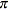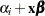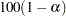The SURVEYLOGISTIC Procedure
 OUTPUT Statement
OUTPUT <OUT=SAS-data-set> <options> < / option> ;

The OUTPUT statement creates a new SAS data set that contains all the variables in the input data set and, optionally, the estimated linear predictors and their standard error estimates, the estimates of the cumulative or individual response probabilities, and the confidence limits for the cumulative probabilities. Formulas for the statistics are given in the section Linear Predictor, Predicted Probability, and Confidence Limits.

If you use the single-trial syntax, the data set also contains a variable named _LEVEL_, which indicates the level of the response that the given row of output is referring to. For example, the value of the cumulative probability variable is the probability that the response variable is as large as the corresponding value of _LEVEL_. For details, see the section OUT= Data Set in the OUTPUT Statement.

The estimated linear predictor, its standard error estimate, all predicted probabilities, and the confidence limits for the cumulative probabilities are computed for all observations in which the explanatory variables have no missing values, even if the response is missing. By adding observations with missing response values to the input data set, you can compute these statistics for new observations, or for settings of the explanatory variables not present in the data, without affecting the model fit.

You can specify the following options in the OUTPUT statement:

LOWER | L=name

names the variable that contains the lower confidence limits for, whereis the probability of the event response if events/trials syntax or the single-trial syntax with binary response is specified;is cumulative probability (that is, the probability that the response is less than or equal to the value of _LEVEL_) for a cumulative model; andis the individual probability (that is, the probability that the response category is represented by the value of _LEVEL_) for the generalized logit model. See the ALPHA= option for information about setting the confidence level.

OUT=SAS-data-set

names the output data set. If you omit the OUT= option, the output data set is created and given a default name by using the DATAconvention.

The statistic options in the OUTPUT statement specify the statistics to be included in the output data set and name the new variables that contain the statistics.

PREDICTED | P=name

names the variable that contains the predicted probabilities. For the events/trials syntax or the single-trial syntax with binary response, it is the predicted event probability. For a cumulative model, it is the predicted cumulative probability (that is, the probability that the response variable is less than or equal to the value of _LEVEL_); and for the generalized logit model, it is the predicted individual probability (that is, the probability of the response category represented by the value of _LEVEL_).

PREDPROBS=(keywords)

requests individual, cumulative, or cross validated predicted probabilities. Descriptions of the keywords are as follows.

INDIVIDUAL | I

requests the predicted probability of each response level. For a response variable Y with three levels, 1, 2, and 3, the individual probabilities are Pr(Y1), Pr(Y2), and Pr(Y3).

CUMULATIVE | C

requests the cumulative predicted probability of each response level. For a response variable Y with three levels, 1, 2, and 3, the cumulative probabilities are Pr(Y1), Pr(Y2), and Pr(Y3). The cumulative probability for the last response level always has the constant value of 1. For generalized logit models, the cumulative predicted probabilities are not computed and are set to missing.

CROSSVALIDATE | XVALIDATE | X

requests the cross validated individual predicted probability of each response level. These probabilities are derived from the leave-one-out principle; that is, dropping the data of one subject and reestimating the parameter estimates. PROC SURVEYLOGISTIC  uses a less expensive one-step approximation to compute the parameter estimates. This option is valid only for binary response models; for nominal and ordinal models, the cross validated probabilities are not computed and are set to missing.

See the section Details of the PREDPROBS= Option at the end of this section for further details.

STDXBETA=name

names the variable that contains the standard error estimates of XBETA (the definition of which follows).

UPPER | U=name

names the variable that contains the upper confidence limits for, whereis the probability of the event response if events/trials syntax or single-trial syntax with binary response is specified;is cumulative probability (that is, the probability that the response is less than or equal to the value of _LEVEL_) for a cumulative model; andis the individual probability (that is, the probability that the response category is represented by the value of _LEVEL_) for the generalized logit model. See the ALPHA= option for information about setting the confidence level.

XBETA=name

names the variable that contains the estimates of the linear predictor, whereis the corresponding ordered value of _LEVEL_.

You can specify the following option in the OUTPUT statement after a slash (/):

ALPHA=value

sets the level of significancefor% confidence limits for the appropriate response probabilities. The valuemust be between 0 and 1. By default,is equal to the value of the ALPHA= option in the PROC SURVEYLOGISTIC  statement, or 0.05 if the ALPHA= option is not specified.Previous Page | Next Page | Top of Page